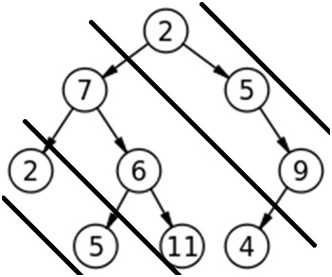# Diagonal Traversal of Binary Tree

In this article, we are going to see how to print diagonal traversal of a binary tree? This problem has been featured in coding round of Amazon.
Submitted by Radib Kar, on February 20, 2019

Problem statement:

Given a binary tree, print the diagonal traversal of the binary tree.

Example:

Consider lines of slope -1 passing between nodes. Given a Binary Tree, print all diagonal elements in a binary tree belonging to same line.In the above example lines of slope -1 are passed between nodes and the diagonal traversal will be: 2 5 9 7 6 11 4 2 5

Solution

Pre-requisite:

Queue q, root of binary tree, Node* temp, Node* temp1

Algorithm:

The algorithm is actually processing the right children and EnQueueing the left children for each parent node. The EnQueued children accts as nodes to be processed for next level.

```FUNCTION diagonalPrint(root)
EnQueue (q, root);
While (q is not empty){
temp=DeQueue(q);
Print temp->data;
temp1=temp;
While (temp1 is not NULL)
IF (temp1->left is not NULL) //if left child exists
EnQueue (q, temp1->left); //enqueue
END IF
temp1=temp1->right; //traverse to right child
IF (temp1 is not NULL)
Print temp1->data;
END IF
END While
END While
END FUNCTION
```

Example with explanation:

Nodes are represented with their respective values for better understanding.

```In main we call diagonalPrint(2)
---------------------------------------------------------

diagonalPrint(2)
q.push(2)
---------------------------------------------------------

1st iteration
temp=2
print 2
temp1=temp//2

////////////////////inner while loop////////////////////
0th iteration
temp1 is not NULL
2->left is not NULL
q.push(2->left) //7
temp1=2->right//5
Print 5
1st iteration
temp1 is not NULL//5
temp1->left is NULL
nothing to push
temp1=temp1->right //9
Print 9
2nd iteration
temp1 is not NULL//9
temp1->left is not NULL
q.push(9->left)//4
temp1=temp1->right //NULL
nothing to print
3rd iteration
temp1 NULL
exit from inner loop
////////////////////inner while loop ends////////////////////

Queue status:
7 4
---------------------------------------------------------

2nd iteration
temp=q.pop() =7
print 7
temp1=temp//7

////////////////////inner while loop////////////////////
0th iteration
temp1 is not NULL
7->left is not NULL
q.push(7->left) //2
temp1=7->right//6
Print 6
1st iteration
temp1 is not NULL//6
temp1->left is not NULL
q.push(6->left) //5
temp1=temp1->right //11
Print 11
2nd iteration
temp1 is not NULL//11
temp1->left is NULL
nothing to push
temp1=temp1->right //NULL
nothing to print
3rd iteration
temp1 NULL
exit from inner loop
////////////////////inner while loop ends////////////////////

Queue status:
4 2 5
---------------------------------------------------------

3rd iteration
temp=q.pop()=4
print 4
temp1=temp//4

////////////////////inner while loop////////////////////
0th iteration
temp1 is not NULL
4->left is NULL
Nothing to push
temp1=4->right//NULL

1st iteration
temp1 is NULL
exit from inner loop
////////////////////inner while loop ends////////////////////

Queue status:
2 5
---------------------------------------------------------

4th iteration
temp=q.pop()=2
print 2
temp1=temp//2

////////////////////inner while loop////////////////////
0th iteration
temp1 is not NULL
2->left is NULL
Nothing to push
temp1=2->right//5
Print 5
1st iteration
temp1 is not NULL//5
temp1->left is NULL
nothing to push
temp1=temp1->right //NULL

2nd iteration
temp1 NULL
exit from inner loop
////////////////////inner while loop ends////////////////////

Queue status:
Empty queue
---------------------------------------------------------
Program ends.
Hence the diagonal traversal is:
2 5 9 7 6 11 4 2 5
```

C++ implementation:

```#include <bits/stdc++.h>
using namespace std;

// tree node is defined
class Node{
public:
int data;
Node *left;
Node *right;
};

void diagonalPrint(Node *root)
{
Node* temp,*temp1;
queue<Node*> q;
q.push(root);
while(!q.empty()){
temp=q.front();
cout<<temp->data<<" ";
q.pop();
temp1=temp;
while(temp1){
//if left child exists EnQueue
if(temp1->left)
q.push(temp1->left);

//process all right children
temp1=temp1->right;
if(temp1)
cout<<temp1->data<<" ";
}
}
}

// creating new node
Node* newnode(int data)
{
Node* node = (Node*)malloc(sizeof(Node));
node->data = data;
node->left = NULL;
node->right = NULL;
return(node);
}

int main()
{
//**same tree is builted as shown in example**
Node *root=newnode(2);

root->left= newnode(7);
root->right= newnode(5);
root->right->right=newnode(9);
root->right->right->left=newnode(4);
root->left->left=newnode(2);
root->left->right=newnode(6);
root->left->right->left=newnode(5);
root->left->right->right=newnode(11);

cout<<"Diagonal traversal of binary tree is :"<<endl;
diagonalPrint(root);

return 0;
}
```

Output

```Diagonal traversal of binary tree is :
2 5 9 7 6 11 4 2 5
```